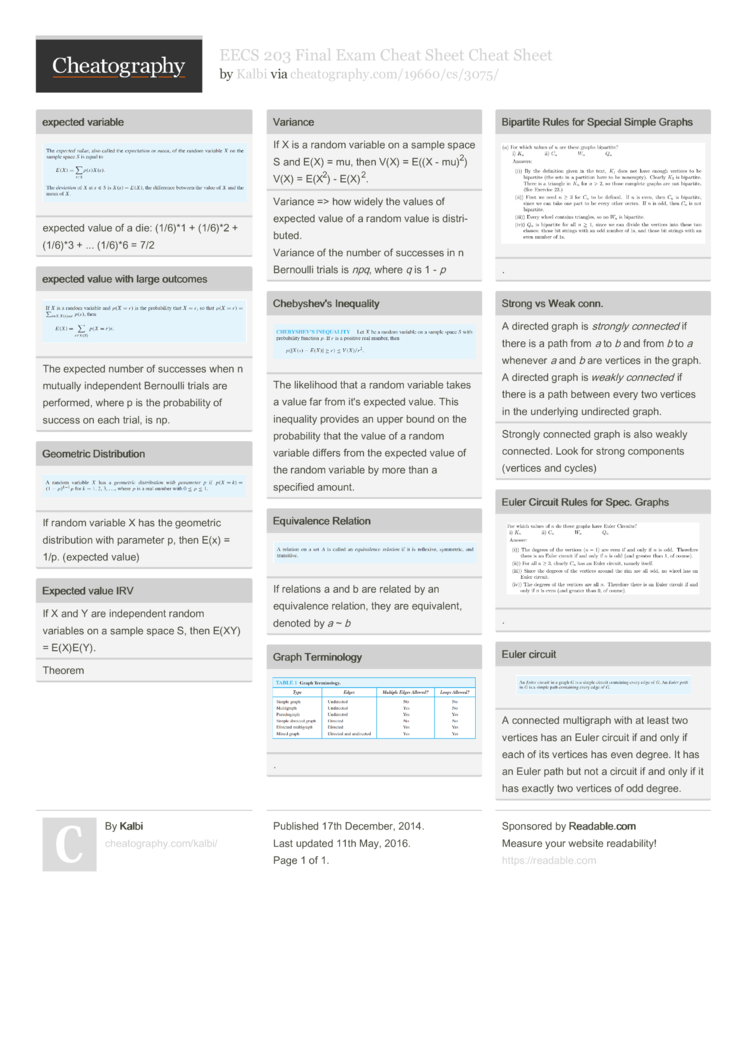# EECS 203 Final Exam Cheat Sheet Cheat Sheet by Kalbi

### expected variableexpected value of a die: (1/6)*1 + (1/6)*2 + (1/6)*3 + ... (1/6)*6 = 7/2

### expected value with large outcomesThe expected number of successes when n mutually indepe­ndent Bernoulli trials are performed, where p is the probab­ility of success on each trial, is np.

### Geometric Distri­butionIf random variable X has the geometric distri­bution with parameter p, then E(x) = 1/p. (expected value)

### Expected value IRV

 If X and Y are indepe­ndent random variables on a sample space S, then E(XY) = E(X)E(Y).
Theorem

### Variance

 If X is a random variable on a sample space S and E(X) = mu, then V(X) = E((X - mu)2) V(X) = E(X2) - E(X)2.
Variance => how widely the values of expected value of a random value is distri­buted.
Variance of the number of successes in n Bernoulli trials is npq, where q is 1 - p

### Chebys­hev's InequalityThe likelihood that a random variable takes a value far from it's expected value. This inequality provides an upper bound on the probab­ility that the value of a random variable differs from the expected value of the random variable by more than a specified amount.

### Equiva­lence RelationIf relations a and b are related by an equiva­lence relation, they are equiva­lent, denoted by a ~ b

### Strong vs Weak conn.

 A directed graph is strongly connected if there is a path from a to b and from b to a whenever a and b are vertices in the graph. A directed graph is weakly connected if there is a path between every two vertices in the underlying undirected graph.
Strongly connected graph is also weakly connected. Look for strong components (vertices and cycles)

### Euler circuitA connected multigraph with at least two vertices has an Euler circuit if and only if each of its vertices has even degree. It has an Euler path but not a circuit if and only if it has exactly two vertices of odd degree.1 Page
//media.cheatography.com/storage/thumb/kalbi_eecs-203-final-exam-cheat-sheet.750.jpg

PDF (recommended)